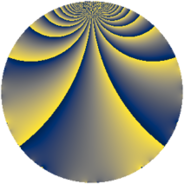# Properties

 Label 1815.2.dLevel $1815$ Weight $2$ Character orbit 1815.d Rep. character $\chi_{1815}(1814,\cdot)$ Character field $\Q$ Dimension $200$ Sturm bound $528$

# Related objects

## Defining parameters

 Level: $$N$$ $$=$$ $$1815 = 3 \cdot 5 \cdot 11^{2}$$ Weight: $$k$$ $$=$$ $$2$$ Character orbit: $$[\chi]$$ $$=$$ 1815.d (of order $$2$$ and degree $$1$$) Character conductor: $$\operatorname{cond}(\chi)$$ $$=$$ $$165$$ Character field: $$\Q$$ Sturm bound: $$528$$

## Dimensions

The following table gives the dimensions of various subspaces of $$M_{2}(1815, [\chi])$$.

Total New Old
Modular forms 288 232 56
Cusp forms 240 200 40
Eisenstein series 48 32 16

## Trace form

 $$200q - 176q^{4} + 12q^{9} + O(q^{10})$$ $$200q - 176q^{4} + 12q^{9} - 2q^{15} + 160q^{16} - 8q^{25} + 32q^{31} - 56q^{34} - 36q^{36} - 38q^{45} + 112q^{49} + 28q^{60} - 168q^{64} + 60q^{69} + 44q^{70} - 6q^{75} + 44q^{81} + 144q^{91} + O(q^{100})$$

## Decomposition of $$S_{2}^{\mathrm{new}}(1815, [\chi])$$ into newform subspaces

The newforms in this space have not yet been added to the LMFDB.

## Decomposition of $$S_{2}^{\mathrm{old}}(1815, [\chi])$$ into lower level spaces

$$S_{2}^{\mathrm{old}}(1815, [\chi]) \cong$$ $$S_{2}^{\mathrm{new}}(165, [\chi])$$$$^{\oplus 2}$$